# PeriodicityThis test lets you check the periodicity. The disc is pierced, the inner surface is fit and the outer surface is submitted to a plane torsion. Thus, the geometry and the limit conditions are periodic.Reference:

3D model with linear hexahedron elements (HE8), computed with CATIA.

##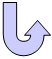Specifications

### Geometry Specifications

 R1 = 0.05 mR2 = 0.1 m h = 0.02 m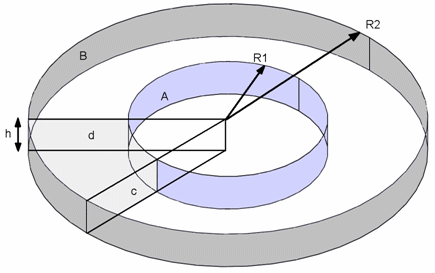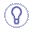For symmetry reasons, only 1/8 of the body is modeled. The given results are the same for 1/8 or for the whole body.

### Analysis Specifications

 Young Modulus (material): E = 200 GPa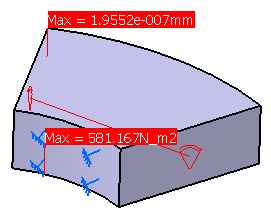Poisson's Ratio (material): ν = 0.3 Mesh Specifications: Linear hexahedron elements (HE8) Mesh size: 10 mm Restraints: Clamp on surface A (inner cylinder) Loads (Surface force density): Tangent to surface B (outer cylinder): 100 N/m2

##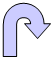Results

The table below presents the analysis results:

 Type of values Full disc model (reference) 1/8 disc model Normalized results (1/8 model / full model) Maximum displacement amplitude (mm) 1.95247 x 10-7 1.9552 x 10-7 1.00140 Maximum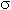Von Mises (N/m2) 586.275 581.167 0.99129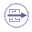To Perform the Test:

The periodicity_full.CATAnalysis document presents a complete analysis, computed with a mesh formed of linear hexahedron elements (HE8).

The periodicity_partial.CATAnalysis document presents a partial analysis, computed with a mesh formed of linear hexahedron elements (HE8).

To compute the case, proceed as follow:

1. Open one of the CATAnalysis documents.

2. Compute the case in the Generative Structural Analysis workbench.

3. Create local sensors (Displacement magnitude and Von Mises stress).